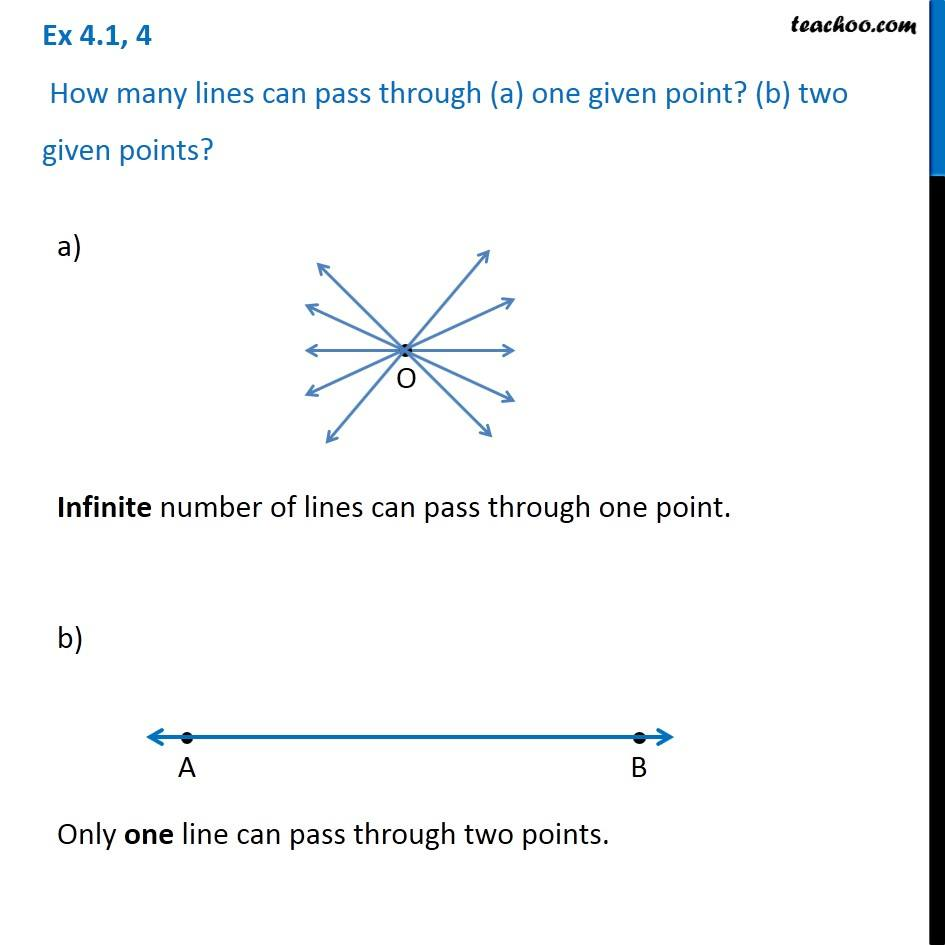1. Chapter 4 Class 6 Basic Geometrical Ideas
2. Concept wise
3. Point, Line, ray, angle

Transcript

Ex 4.1, 4 How many lines can pass through (a) one given point? (b) two given points? a) Infinite number of lines can pass through one point. b) Only one line can pass through two points.